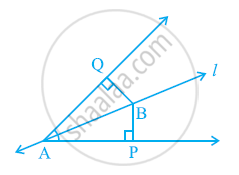Share

# Line l is the bisector of an angle ∠A and B is any point on l. BP and BQ are perpendiculars from B to the arms of ∠A (see the given figure). Show that:- (i) ΔAPB ≅ ΔAQB (ii) BP = BQ or B is equidistant from the arms of ∠A. - CBSE Class 9 - Mathematics

ConceptCriteria for Congruence of Triangles

#### Question

Line l is the bisector of an angle ∠A and B is any point on l. BP and BQ are perpendiculars from B to the arms of ∠A (see the given figure). Show that:-

(i) ΔAPB ≅ ΔAQB

(ii) BP = BQ or B is equidistant from the arms of ∠A.#### Solution

In ΔAPB and ΔAQB,

∠APB = ∠AQB (Each 90º)

∠PAB = ∠QAB (l is the angle bisector of ∠A)

AB = AB (Common)

∴ ΔAPB ≅ ΔAQB (By AAS congruence rule)

∴ BP = BQ (By CPCT)

Or, it can be said that B is equidistant from the arms of ∠A.

Is there an error in this question or solution?

#### APPEARS IN

NCERT Solution for Mathematics Class 9 (2018 to Current)
Chapter 7: Triangles
Ex. 7.10 | Q: 5 | Page no. 119

#### Video TutorialsVIEW ALL 

Solution Line l is the bisector of an angle ∠A and B is any point on l. BP and BQ are perpendiculars from B to the arms of ∠A (see the given figure). Show that:- (i) ΔAPB ≅ ΔAQB (ii) BP = BQ or B is equidistant from the arms of ∠A. Concept: Criteria for Congruence of Triangles.
S